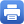Columbia Home
 Home » Programs » Undergraduate Program » Calculus Classes » Accelerated Multivariable CalculusAccelerated Multivariable CalculusPrerequisites: MATH UN1101 (Calculus I) and MATH UN1102 (Calculus II), or equivalents. Textbook: James Stewart, Calculus, Early Transcendentals, 9th edition. Course Description: Vectors in dimensions 2 and 3, vector-valued functions of one variable, scalar-valued functions of several variables, partial derivatives, gradients, optimization, Lagrange multipliers, double and triple integrals, line and surface integrals, vector calculus. Overview and Goals: during this semester you will learn: Multidimensional spaces: coordinate systems, vectors, dot product, cross product, lines and planes. Vector functions: limits, derivatives, and integrals of vector functions; velocity and acceleration. Multivariable differentiation: partial derivatives, directional derivatives, gradients, critical points and the second derivative test, maximum and minimum values, method of Lagrange multipliers. Multivariable integration: double and triple integrals, line and surface integrals, Green’s theorem, Stokes’ theorem, and the divergence theorem. Typical Syllabus: A sample syllabus is available. Help Room for this course (Mathematics UN1205) is located in 333 Milbank Hall, Barnard Campus. In addition to office hours and the Help Room, tutoring services are available. Information on Calculus Classes: For questions not covered here or on the links, contact George Dragomir, the Math Department Calculus Director.Print this page